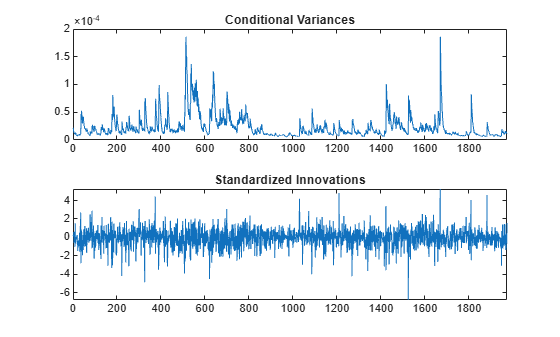# Likelihood Ratio Test for Conditional Variance Models

This example shows how to compare two competing, conditional variance models using a likelihood ratio test.

### Step 1. Load the data and specify a GARCH model.

Load the Deutschmark/British pound foreign exchange rate data included with the toolbox, and convert it to returns. Specify a GARCH(1,1) model with a mean offset to estimate.

```load Data_MarkPound r = price2ret(Data); T = length(r); Mdl = garch('Offset',NaN,'GARCHLags',1,'ARCHLags',1);```

### Step 2. Estimate the GARCH model parameters.

Fit the specified GARCH(1,1) model to the returns series using `estimate`. Return the value of the loglikelihood objective function.

`[EstMdl,~,logL] = estimate(Mdl,r);`
``` GARCH(1,1) Conditional Variance Model with Offset (Gaussian Distribution): Value StandardError TStatistic PValue ___________ _____________ __________ __________ Constant 1.0757e-06 3.5725e-07 3.0112 0.0026021 GARCH{1} 0.80606 0.013274 60.724 0 ARCH{1} 0.15311 0.011532 13.278 3.1257e-40 Offset -6.1314e-05 8.2867e-05 -0.73991 0.45936 ```

The estimation output shows the four estimated parameters and corresponding standard errors. The t statistic for the mean offset is not greater than two in magnitude, suggesting this parameter is not statistically significant.

### Step 3. Fit a GARCH model without a mean offset.

Specify a second model without a mean offset, and fit it to the returns series.

```Mdl2 = garch(1,1); [EstMdl2,~,logL2] = estimate(Mdl2,r);```
``` GARCH(1,1) Conditional Variance Model (Gaussian Distribution): Value StandardError TStatistic PValue __________ _____________ __________ __________ Constant 1.0535e-06 3.5048e-07 3.0058 0.0026487 GARCH{1} 0.80657 0.01291 62.478 0 ARCH{1} 0.15436 0.011574 13.336 1.4293e-40 ```

All the t statistics for the new fitted model are greater than two in magnitude.

### Step 4. Conduct a likelihood ratio test.

Compare the fitted models `EstMdl` and `EstMdl2` using the likelihood ratio test. The number of restrictions for the test is one (only the mean offset was excluded in the second model).

`[h,p] = lratiotest(logL,logL2,1)`
```h = logical 0 ```
```p = 0.4534 ```

The null hypothesis of the restricted model is not rejected in favor of the larger model (`h = 0`). The model without a mean offset is the more parsimonious choice.

### Step 5. Infer the conditional variances and standardized innovations.

Infer and plot the conditional variances and standardized innovations for the fitted model without a mean offset (`EstMdl2`).

```v = infer(EstMdl2,r); inn = r./sqrt(v); figure subplot(2,1,1) plot(v) xlim([0,T]) title('Conditional Variances') subplot(2,1,2) plot(inn) xlim([0,T]) title('Standardized Innovations')```The inferred conditional variances show the periods of high volatility.